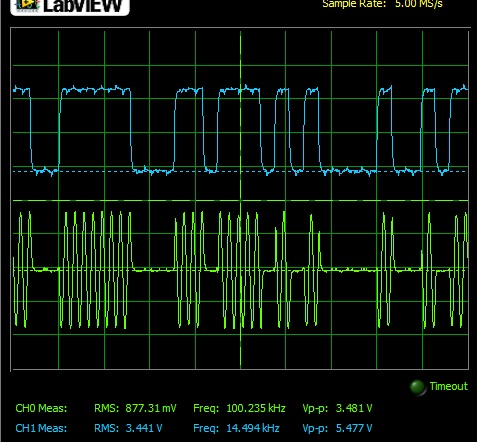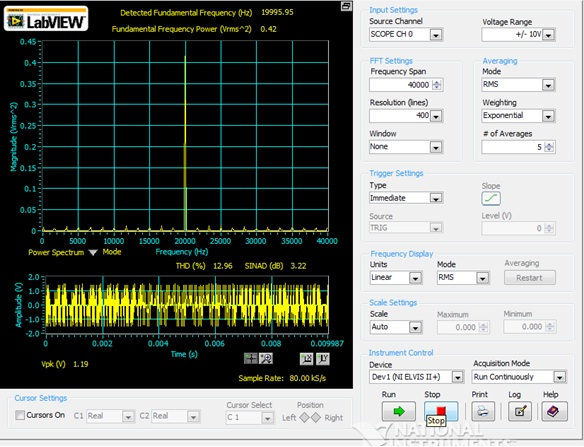Amplitude Shift Keying

Calculations & ObservationsFig.1 Showing ASK modulated signal (green) & digital clock (blue).

In the above Fig.1, the waveforms obtained, represents that when the digital signal is HIGH then the ASK signal has logic ‘1’ i.e. the logic high will show higher frequency of signal with some amplitude but when the digital signal has logic LOW i.e. ‘0’ then the ASK signal will have lower amplitude (0 V).Fig.2 Frequency Spectrum of ASK Signal.

In the fig.2, the frequency spectrum of the ASK signal being modulated using sequence generator has fundamental frequency component present at 19995.95 Hz & the fundamental Frequency Power is 0.42. The THD is 12.96 % & SINAD is 3.22 dB.Fig.3 Power Spectrum in dB scale of ASK signal.

In the Power Spectrum of ASK Signal shown in the Fig.3, theTHD is 11.06 % & SINAD is 3.26 dB. The detected fundamental frequency is at 19996.04 Hz & the fundamental Frequency Power is 0.41.